International
Tables for
Crystallography
Volume B
Reciprocal space
Edited by U. Shmueli

International Tables for Crystallography (2006). Vol. B. ch. 2.4, p. 268   | 1 | 2 |

## Section 2.4.3.5. Determination of phase angles

M. Vijayana* and S. Ramaseshanb

aMolecular Biophysics Unit, Indian Institute of Science, Bangalore 560 012, India, and bRaman Research Institute, Bangalore 560 080, India
Correspondence e-mail:  mv@mbu.iisc.ernet.in

#### 2.4.3.5. Determination of phase angles

| top | pdf |

An important application of anomalous scattering is in the determination of phase angles using Bijvoet differences (Ramachandran & Raman, 1956; Peerdeman & Bijvoet, 1956). From Figs. 2.4.3.2 and 2.4.3.3, we have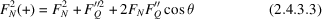and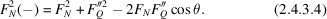Then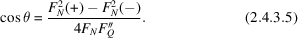In the above equationsmay be approximated to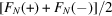. Thencan be evaluated from (2.4.3.5)except for the ambiguity in its sign. Therefore, from Fig. 2.4.3.2, we have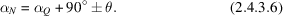The phase angle thus has two possible values symmetrically distributed about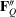. Anomalous scatterers are usually heavy atoms and their positions can most often be determined by Patterson methods.can then be calculated and the two possible values of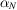for each reflection evaluated using (2.4.3.6).

In practice, the twofold ambiguity in phase angles can often be resolved in a relatively straightforward manner. As indicated earlier, anomalous scatterers usually have relatively high atomic numbers. The `heavy-atom' phases calculated from their positions therefore contain useful information. For any given reflection, that phase angle which is closer to the heavy-atom phase, from the two phases calculated using (2.4.3.6), may be taken as the correct phase angle. This method has been successfully used in several structure determinations including that of a derivative of vitamin B12 (Dale et al., 1963). The same method was also employed in a probabilistic fashion in the structure solution of a small protein (Hendrickson & Teeter, 1981). A method for obtaining a unique, but approximate, solution for phase angles from (2.4.3.6)has also been suggested (Srinivasan & Chacko, 1970). An accurate unique solution for phase angles can be obtained if one collects two sets of intensity data using two different wavelengths which have different dispersion-correction terms for the anomalous scatterers in the structure. Two equations of the type (2.4.3.6)are then available for each reflection and the solution common to both is obviously the correct phase angle. Different types of Patterson and Fourier syntheses can also be employed for structure solution using intensity differences between Bijvoet equivalents (Srinivasan, 1972; Okaya & Pepinsky, 1956; Pepinsky et al., 1957).

An interesting situation occurs when the replaceable atoms in a pair of isomorphous structures are anomalous scatterers. The phase angles can then be uniquely determined by combining isomorphous replacement and anomalous-scattering methods. Such situations normally occur in protein crystallography and are discussed in Section 2.4.4.5.

### ReferencesDale, D., Hodgkin, D. C. & Venkatesan, K. (1963). The determination of the crystal structure of factor V 1a. In Crystallography and crystal perfection, edited by G. N. Ramachandran, pp. 237–242. New York and London: Academic Press.Google ScholarHendrickson, W. A. & Teeter, M. M. (1981). Structure of the hydrophobic protein crambin determined directly from the anomalous scattering of sulphur. Nature (London), 290, 107–113.Google ScholarOkaya, Y. & Pepinsky, R. (1956). New formulations and solution of the phase problem in X-ray analysis for noncentric crystals containing anomalous scatterers. Phys. Rev. 103, 1645–1657.Google ScholarPeerdeman, A. F. & Bijvoet, J. M. (1956). The indexing of reflexions in investigations involving the use of the anomalous scattering effect. Acta Cryst. 9, 1012–1015.Google ScholarPepinsky, R., Okaya, Y. & Takeuchi, Y. (1957). Theory and application of the Ps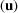function and anomalous dispersion in direct determination of structures and absolute configuration in non-centric crystals. Acta Cryst. 10, 756.Google ScholarRamachandran, G. N. & Raman, S. (1956). A new method for the structure analysis of non-centrosymmetric crystals. Curr. Sci. 25, 348–351.Google ScholarSrinivasan, R. (1972). Applications of X-ray anomalous scattering in structural studies. In Advances in structure research by diffraction methods, Vol. 4, edited by W. Hoppe & R. Mason, pp. 105–197. Braunschweig: Freidr. Vieweg & Sohn; and Oxford: Pergamon Press.Google ScholarSrinivasan, R. & Chacko, K. K. (1970). On the determination of phases of a noncentrosymmetric crystal by the anomalous dispersion method. Z. Kristallogr. 131, 29–39.Google Scholar